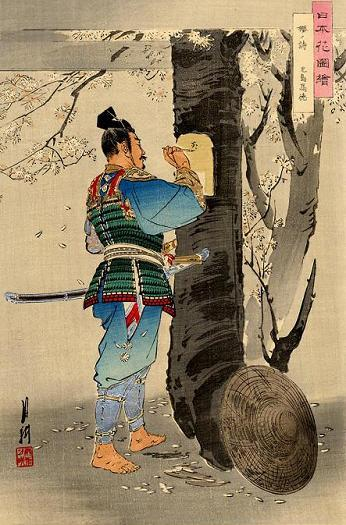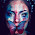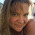## Poet vs. Fighter

I am the poet
You are the fighter
You are the muse
I am the writer

I am the pen
You are the fist
You are the punch
I'm the flick of a wrist

I am the words
You are the blows
You are immovable
I am what flows

I am the stanza
You are the stance
You are the kick
I am the glance

I am the laurel
You are the wreath
You are the bite
I am the teeth

I am the scholar
You are the trained
You are the genuine
I am the feigned

I am the sword
You are the steel
You are the hurt
I am the heal

I am the thought
You are the cause
You are the motion
I am the pause

I am the poem
You are the prose
You are the round
I am the close

I am the match
You're the igniter
You are the poet
I am the fighter

Image: Woodcut by Ogata Gekko, 18951.1.